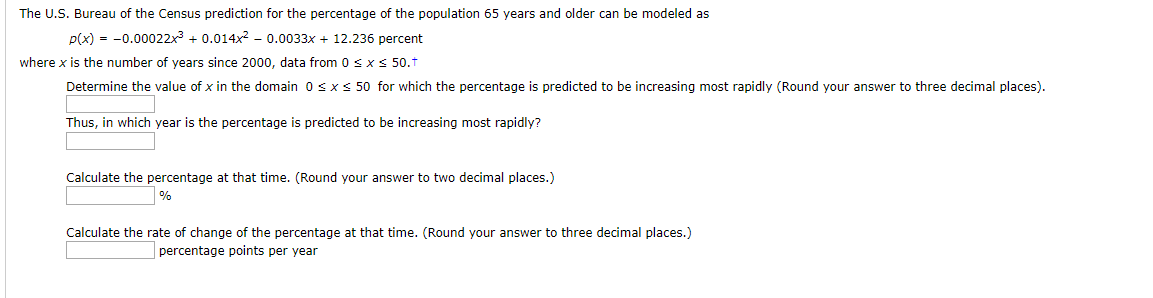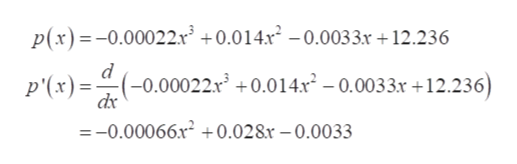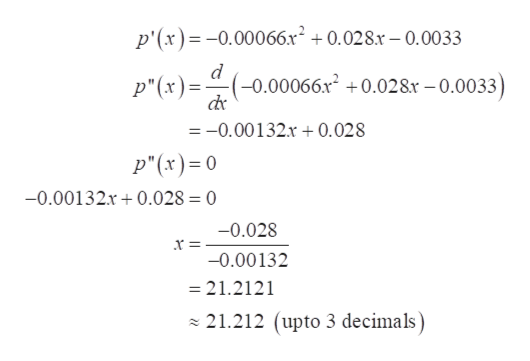# The U.S. Bureau of the Census prediction for the percentage of the population 65 years and older can be modeled asp(x)0.00022x30.014x2- 0.0033x 12.236 percentwhere x is the number of years since 2000, data from 0 s x s 50.tDetermine the value of x in the domain 0x s 50 for which the percentage is predicted to be increasing most rapidly (Round your answer to three decimal places)Thus, in which year is the percentage is predicted to be increasing most rapidly?Calculate the percentage at that time. (Round your answer to two decimal places.)Calculate the rate of change of the percentage at that time. (Round your answer to three decimal places.)percentage points per year

Question
1 viewshelp_outlineImage TranscriptioncloseThe U.S. Bureau of the Census prediction for the percentage of the population 65 years and older can be modeled as p(x)0.00022x30.014x2- 0.0033x 12.236 percent where x is the number of years since 2000, data from 0 s x s 50.t Determine the value of x in the domain 0 x s 50 for which the percentage is predicted to be increasing most rapidly (Round your answer to three decimal places) Thus, in which year is the percentage is predicted to be increasing most rapidly? Calculate the percentage at that time. (Round your answer to two decimal places.) Calculate the rate of change of the percentage at that time. (Round your answer to three decimal places.) percentage points per year fullscreen
check_circle

Step 1

First of all, differentiate p(x) w.r.t. x and get p '(x).

A derivative of a function shows the increasing or decreasing nature of the function.help_outlineImage TranscriptioncloseР(x) --0.00022х +0.014x? 0.0033к + 12.236 d -0.00022x) +0.014x* - 0.0033х +12.236) - -о p'(x) —-0.00066х- +0.028х —0.0033 fullscreen
Step 2

Now, again differentiate p '(x) w.r.t. x to get p '' (x) and equate it to 0 to get the critical points.

The critical point is at x = 21.212help_outlineImage Transcriptionclosep'(x)-0.00066x 0.028.r-0.0033 p"(x)=-(-0.00066x2 +0.028x - 0.0033) =-0.00132.r + 0.028 p"(x)= 0 -0.00132x 0.028 = 0 -0.028 -0.00132 - 21.2121 21.212 (upto 3 decimals) fullscreen
Step 3

Then find the value of p '' (x) at the critical point i.e. x = 21.212.

The value of p '' (x) at the critical point is 0.00000016 and...

### Want to see the full answer?

See Solution

#### Want to see this answer and more?

Solutions are written by subject experts who are available 24/7. Questions are typically answered within 1 hour.*

See Solution
*Response times may vary by subject and question.
Tagged in

### Derivative